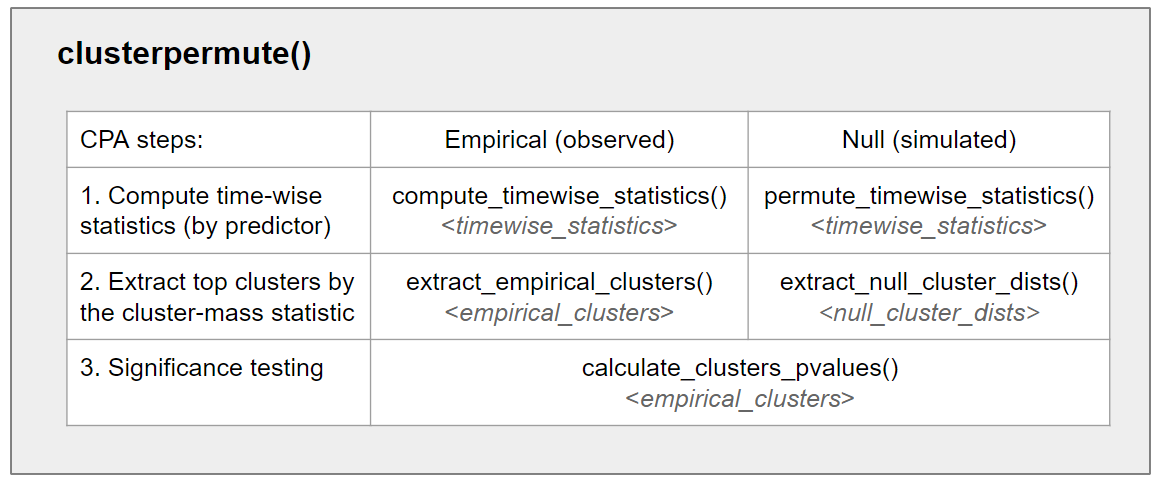# Introduction to jlmerclusterperm

jlmerclusterperm is an interface to regression-based CPAs which supports both wholesale and piecemeal approaches to conducting the test.

## Overview of CPA

Cluster-based permutation analysis (CPA) is a simulation-based, non-parametric statistical test of difference between groups in a time series. It is suitable for analyzing densely-sampled time data (such as in EEG and eye-tracking research) where the research hypothesis is often specified up to the existence of an effect (e.g., as predicted by higher-order cognitive mechanisms) but agnostic to the temporal details of the effect (such as the precise moment of its emergence).

CPA formalizes two intuitions about what it means for there to be a difference between groups:

1. The countable unit of difference (i.e., a cluster) is a contiguous, uninterrupted span of sufficiently large differences at each time point (determined via a threshold).

2. The degree of extremity of a cluster (i.e., the cluster-mass statistic) is a measure that is sensitive to the magnitude of the difference, its variability, and the sample size (e.g., the t-statistic from a regression).

The CPA procedure identifies empirical clusters in a time series data and tests the significance of the observed cluster-mass statistics against bootstrapped permutations of the data. The bootstrapping procedure samples from the “null” (via random shuffling of condition labels), yielding a distribution of cluster-mass statistics emerging from chance. The statistical significance of a cluster is the probability of observing a cluster-mass statistic as extreme as the cluster’s against the simulated null distribution.

## Package designjlmerclusterperm package function design

jlmerclusterperm provides both a wholesale and a piecemeal approach to conducting a CPA. The main workhorse function in the package is clusterpermute(), which is composed of five smaller functions that are called internally in succession. The smaller functions representing the algorithmic steps of a CPA are also exported, to allow more control over the procedure (e.g., for debugging and diagnosing a CPA run).

See the function documentation for more.

## Organization of vignettes

The package vignettes are roughly divided into two groups: topics and case studies. If you are a researcher with data ready for analysis, it is recommended to go through the case studies first and pluck out the relevant bits for your own desired analysis

In the order of increasing complexity, the case studies are:

1. Garrison et al. 2020, which introduces the package’s functions for running a CPA, demonstrating both wholesale and piecemeal approaches. It also compares the use of linear vs. logistic regression for the calculation of timewise statistics.

2. Geller et al. 2020, which demonstrates the use of random effects and regression contrasts in the CPA specification. It also compares the use of t vs. chisq timewise statistics.

3. de Carvalho et al. 2021, which showcases using custom regression contrasts to test the relationship between multiple levels of a factor. It also discusses issues surrounding the complexity of the timewise regression model.

The topics cover general package features:

## Compared to other implementations

There are other R packages for cluster-based permutation analysis, such as clusterperm, eyetrackingR, permuco, and permutes.

Compared to existing implementations, jlmerclusterperm is the only package optimized for CPAs based on mixed-effects regression models, suitable for typical experimental research data with multiple, crossed grouping structures (e.g., subjects and items). It is also the only package with an interface to the individual algorithmic steps of a CPA. Lastly, thanks to the Julia back-end, bootstrapping is fast even for mixed models.